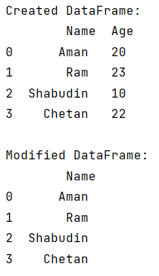# Python pandas DataFrame, is it pass-by-value or pass-by-reference?

Given a Pandas DataFrame, learn with the examples is it pass-by-value or pass-by-reference?
Submitted by Pranit Sharma, on July 01, 2022

Pandas is a special tool that allows us to perform complex manipulations of data effectively and efficiently. Inside pandas, we mostly deal with a dataset in the form of DataFrame. DataFrames are 2-dimensional data structures in pandas. DataFrames consist of rows, columns, and data.

But, In python, we have two types of data types, mutable (which can be updated) like lists, dictionaries, pandas DataFrames, and non-mutable (which cannot be updated) like ints, strings, and tuples.

When we define a variable in python, it acts as an object ad object is a real-world entity whose copies can be made but if it is of a nun-mutable nature, it cannot be updated.

## Problem statement

Whenever we define a function python, we sometimes need some arguments inside it for our operation, when we pass an argument in the form of a variable (object), its copy is passed in place of the parameter defined in the function.

For example, suppose we have a function called hello(x), which has a parameter x, and we have a variable called a=10, here we have a variable whose values is already assigned. Now if we call this function and pass this variable as an argument, a copy of a will be passed and it will be stored in x because of which it looks like we are passing it by reference but we pass the arguments by value.

## Pandas DataFrame: is it pass-by-value or pass-by-reference

If the object is mutable, it can be changed internally and externally, but if the object is non-mutable its original values cannot be updated. Also if we change mutable values inside a function, the changed values will be stored in that local variable and will not change the global variable whose copy was passed and stored in the local variable.

If we want to change the value of a global variable inside a function, we need to make the variable a global variable inside the function using the keyword global and then we can call this function.

Note

To work with pandas, we need to import pandas package first, below is the syntax:

```import pandas as pd
```

Let us understand with the help of an example,

## Python program to demonstrate pass-by-value or pass-by-reference with Pandas DataFrame

```# Importing pandas package
import pandas as pd

# Importing numpy package
import numpy as np

# Creating a DataFrame
df = pd.DataFrame({
'Name':['Aman','Ram','Shabudin','Chetan'],
'Age':[20,23,10,22]
})

# Display DataFrame
print("Created DataFrame:\n",df,"\n")

# defining a function
def function():
global df
df = df.drop('Age',axis=1)

function()

# Display modified DataFrame
print("Modified DataFrame:\n",df)
```

## Output

The output of the above program is: